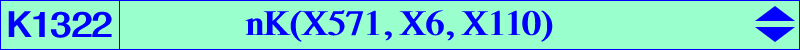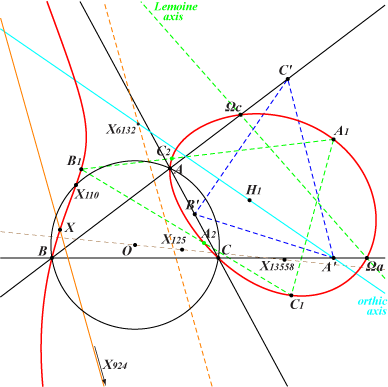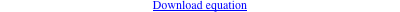too complicated to be written here. Click on the link to download a text file.X(110), X(924), X(53776) Ωa, Ωb, Ωc : centers of the Apollonius circles other points below Geometric properties :K1322 is a circular cubic with singular focus F = X(13558), the circumcircle-inverse of X(125). Its orthic line passes through {924, 6132, 8651, etc} hence its homothetic under h(F, 2) is the real asymptote of K1322. For every point P on K1322, the orthocenter of the pedal triangle of P is a point M on the orthic axis. The isogonal transform of K1322 is nK(X2165, X2165, X523) with singular focus X(125). *** Points on K1322 • A1 on the lines A, X(110) and Ωa, X(924). The points B1, C1 are defined likewise. • A2 on the lines B1, C1 and Ωa, X as below . Its X(571)-isoconjugate is A4. The points B2, C2 and B4, C4 are defined likewise. • A3 on the lines A, X(924) and Ωa, X(110). The points B3, C3 are defined likewise. These three points are the X(571)-isoconjugates of A1, B1, C1.   The triangles ΩaΩbΩc and A2B2C2 are perspective at X on the cubic, where X is the common tangential of X(110), X(924), on the real asymptote. The triangles ABC and A2B2C2 are perspective at Y on the cubic and on the line {110, 924}, {186, 3003}, {523, 687}, {2986, 47348}, {5467, 18879}. Y is now X(53776) in ETC, and then X is the barycentric quotient X(571) ÷ Y. In the figure above, P = A1 with pedal triangle A'B'C' whose orthocenter is H1 on the orthic axis. A' lies on the orthic axis. *** Generalization Let P = p:q:r and L the line with equation p x + q y + r z = 0. The orthocenter of the pedal triangle of a point M lies on L if and only if M lies on a circular circum-cubic K(L) passing through the isogonal conjugate Q of the infinite point of L, a point on the circumcircle. K(L) is a nK if and only if P lies on a nodal cubic (K1) with node G, passing through the midpoints of ABC, X(69), X(1249), X(6389), X(16051). The nodal tangents pass through X(2592), X(2593), on the Kiepert hyperbola and on the line {297,525}. In this case, • the pole Ω of K(L) lies on K429 = psK(X1974, X4, X6), the barycentric product H × K009, • the root R of K(L) lies on a nodal circum-cubic (KR), isotomic transform of K657, with node G, passing through X(6), X(264), X(344), X(1995), X(5958), X(11059), X(34289), X(37800), X(43084), meeting (O) at the same points as pK(X2, X11059), • the singular focus F lies on the circle with center X(26), passing through X(2079). This is the inverse of the nine points circle in (O). Note that F is the inverse of the complement of Q. This circle also contains X(i) for i = 2931, 8428, 10016, 10117, 13558, 14656, 14657, 14667, 14703, 14729, 15959, 19165, 34131, 39828, 39857 and the vertices of the tangential triangle. The anticomplement (K2) of (K1) is a nodal circum-cubic with node G, passing through X(193), X(253), X(393), X(4232), meeting (O) at the same points as pK(X4232, X2). The nodal tangents are obviously the same as those of (K1).